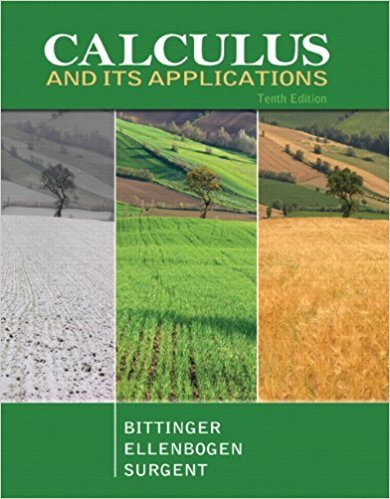×
×

# Solutions for Chapter 3.6: An Economics Application: Elasticity of Demand## Full solutions for Calculus and Its Applications | 10th Edition

ISBN: 9780321694331Solutions for Chapter 3.6: An Economics Application: Elasticity of Demand

Solutions for Chapter 3.6
4 5 0 260 Reviews
11
2
##### ISBN: 9780321694331

Chapter 3.6: An Economics Application: Elasticity of Demand includes 21 full step-by-step solutions. Since 21 problems in chapter 3.6: An Economics Application: Elasticity of Demand have been answered, more than 26129 students have viewed full step-by-step solutions from this chapter. This expansive textbook survival guide covers the following chapters and their solutions. Calculus and Its Applications was written by and is associated to the ISBN: 9780321694331. This textbook survival guide was created for the textbook: Calculus and Its Applications, edition: 10.

Key Calculus Terms and definitions covered in this textbook
• Annual percentage rate (APR)

The annual interest rate

• Average velocity

The change in position divided by the change in time.

• Convenience sample

A sample that sacrifices randomness for convenience

• Deductive reasoning

The process of utilizing general information to prove a specific hypothesis

• Direction of an arrow

The angle the arrow makes with the positive x-axis

• First quartile

See Quartile.

• General form (of a line)

Ax + By + C = 0, where A and B are not both zero.

• Independent events

Events A and B such that P(A and B) = P(A)P(B)

• Main diagonal

The diagonal from the top left to the bottom right of a square matrix

• Ordered pair

A pair of real numbers (x, y), p. 12.

• Piecewise-defined function

A function whose domain is divided into several parts with a different function rule applied to each part, p. 104.

• Plane in Cartesian space

The graph of Ax + By + Cz + D = 0, where A, B, and C are not all zero.

• Product of matrices A and B

The matrix in which each entry is obtained by multiplying the entries of a row of A by the corresponding entries of a column of B and then adding

• Quartic function

A degree 4 polynomial function.

• Randomization

The principle of experimental design that makes it possible to use the laws of probability when making inferences.

• Rational numbers

Numbers that can be written as a/b, where a and b are integers, and b ? 0.

• Symmetric property of equality

If a = b, then b = a

• Unit vector

Vector of length 1.

• Vertex form for a quadratic function

ƒ(x) = a(x - h)2 + k

• Zero vector

The vector <0,0> or <0,0,0>.

×